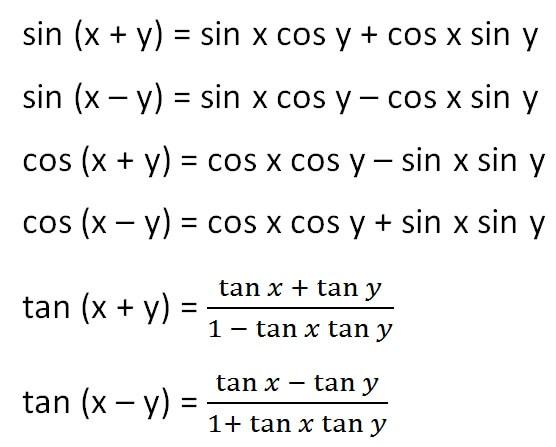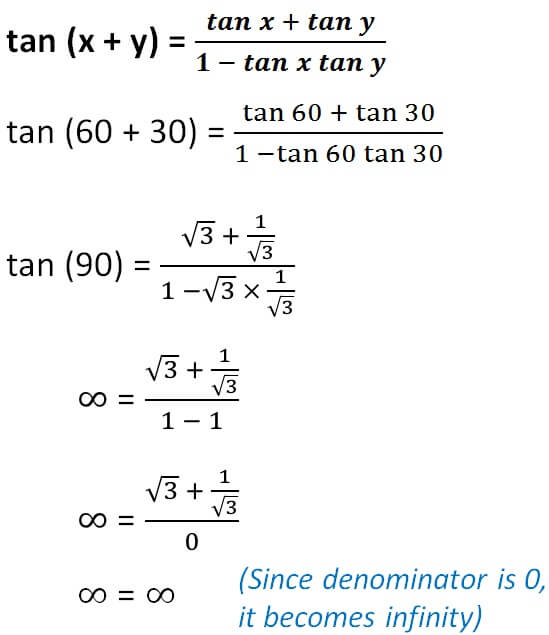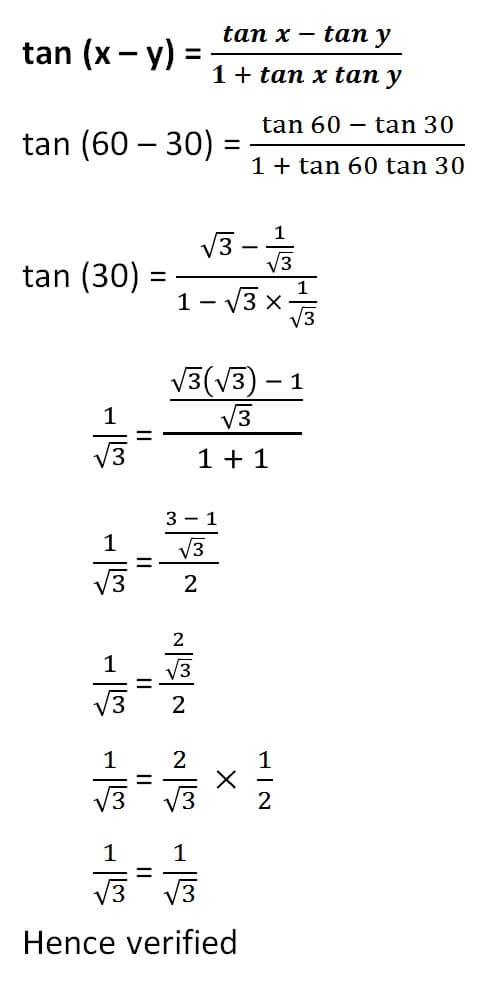(x + y) formula

Chapter 3 Class 11 Trigonometric Functions
Concept wise## Let’s see how we can learn it

1.In sin , we have sin cos.

In cos , we have cos cos, sin sin

In tan , we have sum above, and product below

2.For sin (x + y), we have + sign on right..

For sin (x – y), we have – sign on right right.

For cos, it becomes opposite

For cos (x + y), we have – sign on right..

For cos (x – y), we have + sign on right right.

For tan (x + y), numerator is positive & denominator is negative

For tan (x – y), numerator is negative & denominator is positive

Let’s take x = 60°, y = 30° and verify

### sin (x + y) = sin x cos y + cos x sin y

sin (60° + 30°) = sin 60° cos 30° + cos 60° sin 30°

sin (90°) = (√3/2) × (√3/2) + (1/2)  × (1/2)

1 = 3/4 + 1/4

1 = 4/4

1 = 1

Hence verified

### sin (x – y) = sin x cos y – cos x sin y

sin (60° – 30°) = sin 60° cos 30° – cos 60° sin 30°

sin (30°) = (√3/2) × (√3/2) + (1/2)  × (1/2)

1/2 = 3/4 – 1/4

1/2 = 2/4

1/2 = 1/2

Hence verified

### cos (x + y) = cos x cos y – sin x sin y

cos (60° + 30°) = cos 60° cos 30° – sin 60° sin 30°

cos (90°) = (1/2) × (√3/2) – (√3/2) × (1/2)

0 = √3/4 – √3/4

0 = 0

Hence verified

### cos (x – y) = cos x cos y + sin x sin y

cos (60° – 30°) = cos 60° cos 30° + sin 60° sin 30°

cos (30°) = (1/2) × (√3/2) +  (√3/2) × (1/2)

√3/2 = √3/4 +  √3/4

√3/2 = 2 × √3/4

√3/2 = √3/2

Hence verifiedLearn in your speed, with individual attention - Teachoo Maths 1-on-1 Class

### Transcript

Let’s see how we can learn it 1.In sin, we have sin cos. In cos, we have cos cos, sin sin In tan, we have sum above, and product below 2.For sin (x + y), we have + sign on right.. For sin (x – y), we have – sign on right right. For cos, it becomes opposite For cos (x + y), we have – sign on right.. For cos (x – y), we have + sign on right right. For tan (x + y), numerator is positive & denominator is negative For tan (x – y), numerator is negative & denominator is positive Let’s take x = 60°, y = 30° and verify sin (x + y) = sin x cos y + cos x sin y sin (60° + 30°) = sin 60° cos 30° + cos 60° sin 30° sin (90°) = (√3/2) × (√3/2) + (1/2) × (1/2) 1 = 3/4 + 1/4 1 = 4/4 1 = 1 Hence verified sin (x – y) = sin x cos y – cos x sin y sin (60° – 30°) = sin 60° cos 30° – cos 60° sin 30° sin (30°) = (√3/2) × (√3/2) + (1/2) × (1/2) 1/2 = 3/4 – 1/4 1/2 = 2/4 1/2 = 1/2 Hence verified cos (x + y) = cos x cos y – sin x sin y cos (60° + 30°) = cos 60° cos 30° – sin 60° sin 30° cos (90°) = (1/2) × (√3/2) – (√3/2) × (1/2) 0 = √3/4 – √3/4 0 = 0 Hence verified cos (x – y) = cos x cos y + sin x sin y cos (60° – 30°) = cos 60° cos 30° + sin 60° sin 30° cos (30°) = (1/2) × (√3/2) + (√3/2) × (1/2) √3/2 = √3/4 + √3/4 √3/2 = 2 × √3/4 √3/2 = √3/2 Hence verified